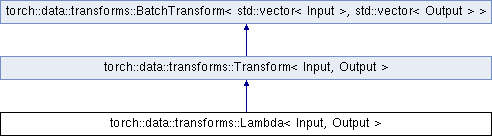Caffe2 - C++ API A deep learning, cross platform ML framework
torch::data::transforms::Lambda< Input, Output > Class Template Reference
Inheritance diagram for torch::data::transforms::Lambda< Input, Output >:## Public Types

using FunctionType = std::function< Output(Input)>Public Types inherited from torch::data::transforms::Transform< Input, Output >
using InputType = Input

using OutputType = OutputPublic Types inherited from torch::data::transforms::BatchTransform< std::vector< Input >, std::vector< Output > >
using InputBatchType = std::vector< Input >

using OutputBatchType = std::vector< Output >

## Public Member Functions

Lambda (FunctionType function)
Constructs the `Lambda` from the given `function` object.

OutputType apply (InputType input) override
Applies the user-provided function object to the `input`.Public Member Functions inherited from torch::data::transforms::Transform< Input, Output >
std::vector< Output > apply_batch (std::vector< Input > input_batch) override
Applies the `transformation` over the entire `input_batch`.

## Detailed Description

### template<typename Input, typename Output = Input> class torch::data::transforms::Lambda< Input, Output >

Definition at line 36 of file lambda.h.

The documentation for this class was generated from the following file:
• torch/csrc/api/include/torch/data/transforms/lambda.h